# NCERT Solutions for Class 8 Maths Chapter 5 Data Handling| PDF Download

You will get Chapter 5 Data Handling NCERT Solutions for Class 8 Maths here that will help in completing the homework and understanding the key concepts of the chapter. You can download PDF of Class 8 Maths Chapter 5 Data Handling NCERT Solutions which are accurate and detailed and as per the latest syllabus released by CBSE.

Studyrankers subject matter experts have taken every while preparing these Chapter 5 Data Handling NCERT Solutions that help in solving the difficulties that lie ahead with ease. Students can develop their understanding of the chapter and obtain maximum marks in the exams.Page No: 76

Exercise 5.1

1. For which of these would you use a histogram to show the data?
(a) The number of letters for different areas in a postman’s bag.
(b) The height of competitors in an athletics meet.
(c) The number of cassettes produced by 5 companies.
(d) The number of passengers boarding trains from 7:00 a.m. to 7:00 p.m. at a station.
Give reasons for each.

Since, Histogram is a graphical representation of data, if data represented in manner of class-interval.
Therefore, for case (b) and (d), we would use a histogram to show the data, because in these cases, data can be divided into class-intervals.
In case (b), a group of competitiors having different heights in an athletics meet.
In case (d), the number of passengers boarding trains in an interval of one hour at a station.

2. The shoppers who come to a departmental store are marked as: man (M), woman (W), boy (B) or girl (G). The following list gives the shoppers who came during the first hour in the morning.
W W W G B W W M G G M M W W W W G B M W B G G M W W M M W W W M W B W G M W W W W G W M M W M W G W M G W M M B G G W
Make a frequency distribution table using tally marks.
Draw a bar graph to illustrate it.

The frequency distribution table is as follows: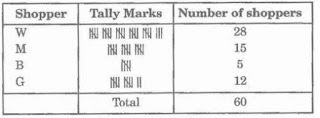The illustration of data by bar-graph is as follows:3. The weekly wages (in ₹) of 30 workers in a factory are:
830, 835, 890, 810, 835, 836, 869, 845, 898, 890, 820, 860, 832, 833, 855, 845, 804, 808, 812, 840, 885, 835, 835, 836, 878, 840, 868, 890, 806, 840
Using tally marks, make a frequency table with intervals as 800 – 810, 810 – 820 and so on.

The representation of data by frequency distribution table using tally marks is as follows: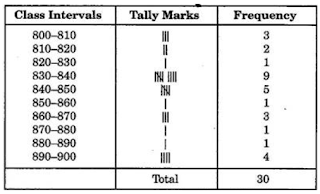4. Draw a histogram for the frequency table made for the data in Question 3 and answer the following questions.
(i) How many workers earn ₹850 and more?
(ii) How many workers earn less than ₹850?

830 – 840 group has the maximum number of workers.

(i) 10 workers can earn more than ₹850.
(ii) 20 workers earn less than ₹850.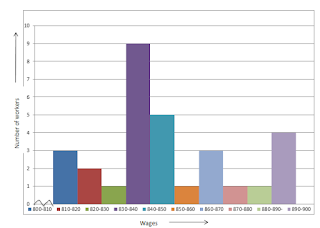5. The number of hours for which students of a particular class watched television during holidays is shown through the given graph.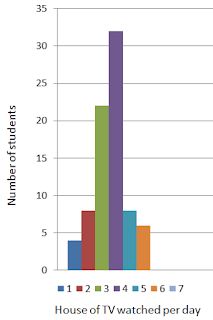(i) For how many hours did the maximum number of students watch T.V.?
(ii) How many students watched TV for less than 4 hours?
(iii) How many students spent more than 5 hours in watching TV?

(i) The maximum number of students watched T.V. for 4 – 5 hours.
(ii) 34 students watched T.V. for less than 4 hours.
(iii) 14 students spent more than 5 hours in watching T.V.

Exercise 5.2

1. A survey was made to find the type of music that a certain group of young people liked in a city. Adjoining pie chart shows the findings of this survey.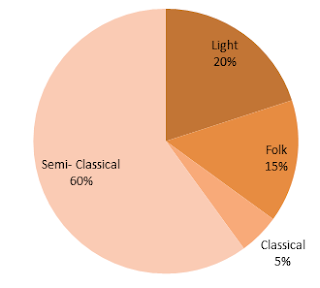From this pie chart, answer the following:
(i) If 20 people liked classical music, how many young people were surveyed?
(ii) Which type of music is liked by the maximum number of people?
(iii) If a cassette company were to make 1000 CD’s, how many of each type would they make?

(i) 10% represents 20 people.
Therefore 100% represents = (100×20)/10 = 200 people
Hence, 200 people were surveyed.

(ii) Light music is liked by the maximum number of people.

(iii) CD’s of classical music = (10 × 1000)/100 = 100
CD’s of semi-classical music = (20×1000)/100 = 200
CD’s of light music = (40×1000)/100 = 400
CD’s of folk music = (30 × 1000)/100 = 300

2. A group of 360 people were asked to vote for their favourite season from the three seasons rainy, winter and summer.
(i) Which season got the most votes?
(ii) Find the central angle of each sector.
(iii) Draw a pie chart to show this information.
 Season Number of Votes Summer 90 Rainy 120 Winter 150

(i) Winter season got the most votes.

(ii) Central angle of summer season = (90° × 360°)/360° = 90°
Central angle of rainy season = (120° ×360°)/ 360° = 120°
Central angle of winter season = (150° ×360°)/360° = 150°(iii)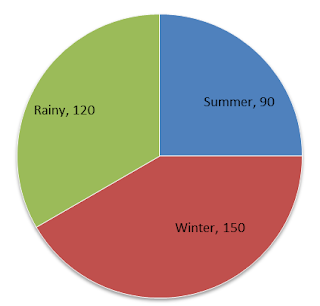3. Draw a pie chart showing the following information. The table shows the colours preferred by a group of people.
 Colours Number of People Blue 18 Green 9 Red 6 Yellow 3 Total 36

Here, central angle = 360° and total number of people = 36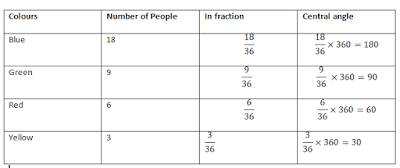4. The adjoining pie chart gives the marks scored in an examination by a student in Hindi, English, Mathematics, Social Science and Science. If the total marks obtained by the students were 540, answer the following questions:(i) In which subject did the student score 105 marks?
(Hint: for 540 marks, the central angle = 360°. So, for 105 marks, what is the central angle?)
(ii) How many more marks were obtained by the student in Mathematics than in Hindi?
(iii) Examine whether the sum of the marks obtained in Social Science and Mathematics is more than that in Science and Hindi.
(Hint: Just study the central angles)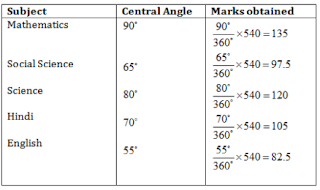(i) The student scored 105 marks in Hindi.

(ii) Marks obtained in Mathematics = 135
Marks obtained in Hindi = 105
Difference = 135 – 105 = 30
Thus, 30 more marks were obtained by the student in Mathematics than in Hindi.

(iii) The sum of marks in Social Science and Mathematics = 97.5 + 135 = 232.5
The sum of marks in Science and Hindi = 120 + 105 = 225
Yes, the sum of the marks in Social Science and Mathematics is more than that in Science and Hindi.

5. The number of students in a hostel, speaking different languages is given below.
 Language Hindi English Marathi Tamil Bengali Total Number of Students 40 12 9 7 4 72
Display the data in a pie chart.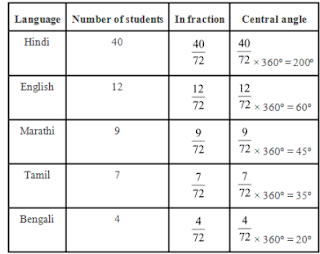Pie chart at above given data is as follows.Exercise 5.3

1. List the outcomes you can see in these experiments.
(a) Spinning a wheel(b) Tossing two coins together

(a) There are four letters A, B, C and D in a spinning wheel. So there are 4 outcomes.
(b) When two coins are tossed together. There are four possible outcomes HH, HT, TH, TT.
(Here HT means head on first coin and tail on second coin and so on.)

2. When a die is thrown, list the outcomes of an event of getting:
(i) (a) a prime number
(b) not a prime number
(ii) (a) a number greater than 5
(b) a number not greater than 5

(i) (a) Outcomes of event of getting a prime number are 2, 3 and 5.
(b) Outcomes of event of not getting a prime number are 1, 4 and 6.

(ii) (a) Outcomes of event of getting a number greater than 5 is 6.
(b) Outcomes of event of not getting a number greater than 5 are 1, 2, 3, 4 and 5.

3. Find the:
(a) Probability of the pointer stopping on D in (Question 1 (a)).
(b) Probability of getting an ace from a well shuffled deck of 52 playing cards.
(c) Probability of getting a red apple. (See figure below)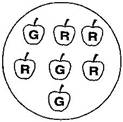(a) In a spinning wheel, there are five pointers A, A, B, C, D. So there are five outcomes. Pointer stops at D which is one outcome.So the probability of the pointer stopping on D = 15

(b) There are 4 aces in a deck of 52 playing cards. So, there are four events of getting an ace.
So, probability of getting an ace = 4/52 = 1/13

(c) Total number of apples = 7
Number of red apples = 4
Probability of getting red apple = 4/7

4. Numbers 1 to 10 are written on ten separate slips (one number on one slip), kept in a box and mixed well. One slip is chosen from the box without looking into it. What is the probability of:
(i) getting a number 6.
(ii) getting a number less than 6.
(iii) getting a number greater than 6.
(iv) getting a 1-digit number.

(i) Outcome of getting a number 6 from ten separate slips is one.
Therefore, probability of getting a number 6 = 1/10

(ii) Numbers less than 6 are 1, 2, 3, 4 and 5 which are five. So there are 5 outcomes.
Therefore, probability of getting a number less than 6 = 5/10 = 1/2

(iii) Number greater than 6 out of ten that are 7, 8, 9, 10. So there are 4 possible outcomes.
Therefore, probability of getting a number greater than 6 ⇒ 4/10 = 2/5

(iv) One digit numbers are 1, 2, 3, 4, 5, 6, 7, 8, 9 out of ten.
Therefore, probability of getting a 1-digit number = 9/10

5. If you have a spinning wheel with 3 green sectors, 1 blue sector and 1 red sector, what is the probability of getting a green sector? What is the probability of getting a none-blue sector?

There are five sectors. Three sectors are green out of five sectors.
Therefore, probability of getting a green sector = 3/5
There is one blue sector out of five sectors.
Non-blue sectors = 5 - 1 = 4 sectors
Therefore, probability of getting a non-blue sector = 4/5

6. Find the probability of the events given in Question 2.

When a die is thrown, there are total six outcomes, i.e., 1, 2, 3, 4, 5 and 6.

(i) (a) 2, 3, 5 are prime numbers. So there are 3 outcomes out of 6.
Therefore, probability of getting a prime number = 3/6 = 1/2

(b) 1, 4, 6 are not the prime numbers. So there are 3 outcomes out of 6.
Therefore, probability of getting a prime number = 3/6 = 1/2

(ii) (a) Only 6 is greater than 5. So there is one outcome out of 6.
Therefore, probability of getting a number greater than 5 = 1/6

(b) Numbers not greater than 5 are 1, 2, 3, 4 and 5. So there are 5 outcomes out of 6.
Therefore, probability of not getting a number greater than 5 = 5/6

Go Back To NCERT Solutions for Class 8 Maths

## NCERT Solutions for Class 8 Maths Chapter 5 Data Handling

Chapter 5 Class 8 Maths NCERT Solutions will help in learning how to solve a questions efficiently. The numerical information is called data which can be arranged and presented by grouped frequency distribution. We will discuss about various type of graphs and probability.

• A Pictograph: Pictorial representation of data using symbols.

• A bar graph: A display of information using bars of uniform width, their heights being proportional to the respective values.

• Double Bar Graph: A bar graph showing two sets of data simultaneously. It is useful for the comparison of the data.

• Frequency is the number of times a particular entry occurs.

• Histogram is a special type of bar graph in which the class intervals are shown on the horizontal axis and heights of the bars correspond to the frequency of the class.

• A circle graph or a pie chart shows the relation ship between a whole and its parts.

• Probability of an event = Number of favourable outcomes / Total Number of Outcomes.

We have also provided exercisewise Class 8 NCERT Solutions below that an individual can check to improve their problem solving skills.

On this page you will get detailed and accurate Chapter 5 Data Handling Class 8 NCERT Solutions which will clear your doubts easily.

### NCERT Solutions for Class 8 Maths Chapters:

 Chapter 1 Rational Numbers Chapter 2 Linear Equations in Variable Chapter 3 Understanding Quadrilaterals Chapter 4 Practical Geometry Chapter 6 Square and Square Roots Chapter 7 Cube and Cube Roots Chapter 8 Comparing Quantities Chapter 9 Algebraic Expressions and Identities Chapter 10 Visualizing Solid Shapes Chapter 11 Mensuration Chapter 12 Exponents and Powers Chapter 13 Direct and Inverse Proportions Chapter 14 Factorization Chapter 15 Introduction to Graphs Chapter 16 Playing with Numbers

FAQ on Chapter 5 Data Handling

#### How many exercises are there in Chapter 5 Data Handling?

There are total 3 exercises in the Chapter 5 which will improve problem skills and understand all the important points of the chapter.  A student will gain confidence that is going to help them in exams by solving these Class 8 Maths NCERT Solutions.

#### A bag contains 3 blue and 2 red balls. A ball is drawn at random. What is the probability of drawing a red ball?

2/5 is the probability of drawing a red ball.

#### When a die is thrown, list the outcomes of an event of getting a number more than 3.

∵ Numbers more than 3 are 4, 5, and 6.
∴ Number of outcomes = 3
∴ Probability of getting a number greater than 3 = 3/6 or 1/2.

#### What is the difference between the highest and the lowest values of observations in a data?

Range of the data.